# Logical Reasoning for Freshers - Set 1

1)   In a certain code language, ‘it be pee’ means ‘dogs are blue’, ‘sik hee’ means ‘large horses’ and ‘pee mit hee’ means ‘horses are pigs’.

How is ‘pigs are large horses’ written in this code?

a. Mit pee sik hee
b. Sik it pee be
c. Cannot be determined
d. None of these
 Answer  Explanation ANSWER: Mit pee sik hee Explanation: In the second and third statements, the common code is ‘hee’ and the common word is ‘horses’. So, ‘hee’ means ‘horses’.Thus, ‘sik’ means ‘large’ from second statement.In first and third statements, the common code is ‘pee’ and common word is ‘are’. So, ‘pee’ means ‘are’.Thus, ‘mit’ means ‘pigs’ from third statement.So, the code is ‘Mit pee sik hee’

2)   In a certain code language, ‘it be pee’ means ‘dogs are blue’, ‘sik hee’ means ‘large horses’ and ‘pee mit hee’ means ‘horses are pigs’.

How is ‘pig’ written in this code?

a. Hee
b. Pee
c. Sik
d. Mit
 Answer  Explanation ANSWER: Mit Explanation: In the second and third statements, the common code is ‘hee’ and the common word is ‘horses’. So, ‘hee’ means ‘horses’.Thus, ‘sik’ means ‘large’ from second statement.In first and third statements, the common code is ‘pee’ and common word is ‘are’. So, ‘pee’ means ‘are’.Thus, ‘mit’ means ‘pigs’ from third statement.

• In a tournament, Pool A and Pool B have seven teams each.
• In each pool, every team plays one match with every other team.
• Two points are awarded for a win, one point for a tie and zero for a loss. At the end of the pool stage, the top three teams in terms of the points scored, advance to the next round called the super stage.
• In the super stage, all the teams start at zero points and the three teams that advance from Pool A play one match each against the three teams that advanced from Pool B.
• Points are awarded as in the pool stage.
• At the end of the matches, the top four teams in terms of the points scored advance to the semi-finals and the winners of the semi-finals play the finals.
• If two or more teams end up with the same number of points at the end of the pool stage or the super stage, the team with better net run rate is placed higher.
What is the minimum no. of matches won by a team that reaches the finals?

a. 1
b. 2
c. 3
d. 4
 Answer  Explanation ANSWER: 1 Explanation: To find minimum no. of matches won, consider the case where all matches in first two stages are drawn and teams advance through net run rate.Pool matches - all matches drawn. Teams advances because of the net run rate. No team won any match yet advanced legally.Super stage - ALL matches DRAWN. Teams advance because of net run rate.Pool & Super stage is over without a single win.Now for any team to reach the finals, it must win the semi-final.So, a team can reach finals with just that one win in Semi-finals.

4)   Choose the number which is different from others in the group

a. 263
b. 111
c. 242
d. 551
e. 383
 Answer  Explanation ANSWER: 383 Explanation: In all other numbers, the middle digit is equal to the product of the other two digits.(263) - 2 x 3 = 6(111) – 1 x 1 = 1(242) – 2 x 2 = 4(551) – 5 x 1 = 5(383) – 3 x 3 = 9, but the number given is 383.

5)   Choose the number which is different from others.

a. 13
b. 17
c. 23
d. 63
e. 71
 Answer  Explanation ANSWER: 63 Explanation: Except 63, all other numbers are prime numbers.

• Six people Nitro, Nemesis, Nick Fury, Viper, Kronos and Hulk are attending a hexagonal table conference.
• All the sides of the hexagon table so formed are of same length.
• Nitro is not adjacent to Nemesis or Nick Fury, Viper is not adjacent to Nick Fury or Kronos.
• Nemesis and Nick Fury are adjacent, Hulk is in the middle of Viper and Nick Fury.
If one neighbor of Nitro is Viper, who is the other one?

a. Nemesis
b. Nick Fury
c. Kronos
d. Hulk
 Answer  Explanation ANSWER: Kronos Explanation: Hulk is in the middle of Viper and Nick Fury.Nemesis and Nick Fury are adjacent.Nitro is not adjacent to Nick Fury.Thus, two arrangements are possible.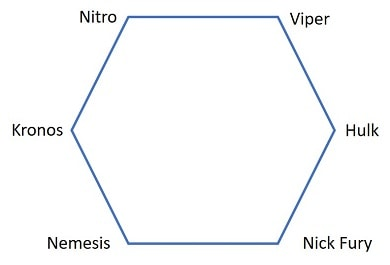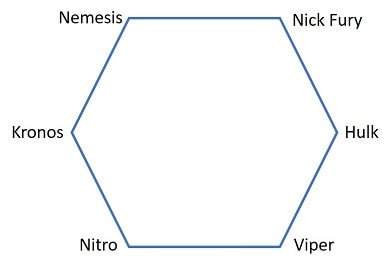Viper and Kronos are neighbors of Nitro.

• Six people Nitro, Nemesis, Nick Fury, Viper, Kronos and Hulk are attending a hexagonal table conference.
• All the sides of the hexagon table so formed are of same length.
• Nitro is not adjacent to Nemesis or Nick Fury, Viper is not adjacent to Nick Fury or Kronos.
• Nemesis and Nick Fury are adjacent, Hulk is in the middle of Viper and Nick Fury.
Who is placed exactly opposite to Kronos?

a. Nemesis
b. Nick Fury
c. Viper
d. Hulk
 Answer  Explanation ANSWER: Hulk Explanation: Hulk is in the middle of Viper and Nick Fury.Nemesis and Nick Fury are adjacent.Nitro is not adjacent to Nick Fury.Thus, two arrangements are possible.Hulk is exactly opposite to Kronos.

8)   In the following question, various terms of an alphabet series are given with one or more missing terms as shown by (?). Choose the missing term out of the given alternatives.

DEMONETIZATION, EMONETIZATIO, MONETIZATI, ONETIZAT,  ?

a. NETIZ
b. NETIZAT
c. ETIZAT
d. NETIZA
 Answer  Explanation ANSWER: NETIZA Explanation: In this series, one letter from the beginning and one letter from the end are removed to obtain the next term.This pattern is repeated here.So, the next term should be ONETIZAT → NETIZA

9)   In the following question, various terms of an alphabet series are given with one or more missing terms as shown by (?). Choose the missing term out of the given alternatives.

IJK, NOP, STU, ?

a. ZYX
b. XYZ
c. WXY
d. YZA
 Answer  Explanation ANSWER: XYZ Explanation: The pattern here is +3i.e. (I → J → K ) __+3__ (N → O → P ) __+3__ (S → T → U ) __+3__ (X → Y → Z )So, the next term should be XYZ.

10)   In the question below is given a statement followed by two courses of action numbered I and II. You have to assume everything in the statement to be true and based on the information given in the statement, decide which of the suggested courses of action logically follow(s) for pursuing.

(A) If only I follows
(B) If only II follows
(C) If either I or II follows
(D) If neither I nor II follow
(E) If both I and II follow.

Statement - Recent protests by the mill-workers has led to a lock down. The business cannot remain profitable without cooperation between the owners and mill-workers.

Courses of Action - I. The mill should be closed.

II. The workers should cooperate with the owners.

III. The owners should talk with the workers about the problems and try to reach a mutually agreeable solution.

a. None follow
b. All follow
c. Only II and III follow
d. Neither I nor II follows
e. Only I and II follow
 Answer  Explanation ANSWER: Only II and III follow Explanation: Here, the statements II and III both together follow as they convey the solution to the above problem. The owners and workers must come together to solve the problem.So, both II and III follow.

11)   In the question below is given a statement followed by two courses of action numbered I and II. You have to assume everything in the statement to be true and based on the information given in the statement, decide which of the suggested courses of action logically follow(s) for pursuing.

(A) If only I follows
(B) If only II follows
(C) If either I or II follows
(D) If neither I nor II follow
(E) If both I and II follow.

Statement - Group of Naxalites armed with weapons were caught by the courageous villagers.

Courses of Action - I. Heavy weapons should be provided to the villagers.

II. The villagers should be rewarded for their courage.

a. Only I follows
b. Only II follows
c. Either I or II follows
d. Neither I nor II follow
e. Both I and II follow
 Answer  Explanation ANSWER: Only II follows Explanation: Providing heavy weapons is not a practical option. However, villagers should be rewarded for their courage in the face of difficulty.Thus, only II follows.

12)   In the following question, a statement/group of statements is given followed by some conclusions. Without resolving anything yourself, choose the conclusion which logically follows from the statement(s).

A man our village has two wives. No one else in the village has two wives.

a. All men in the village have one wife.
b. Every man in the village is married.
c. Some of the men in the village are unmarried.
d. Only one man in the village married twice.
 Answer  Explanation ANSWER: Only one man in the village married twice. Explanation: No explanation is available for this question!

13)   In the following question, a statement/group of statements is given followed by some conclusions. Without resolving anything yourself, choose the conclusion which logically follows from the statement(s).

Most celebrities like Amar are liars.

a. Amar is a celebrity.
b. Those who do not tell lies are not celebrities.
c. Some celebrities do not tell lies.
d. You can only become a celebrity by telling lies.
 Answer  Explanation ANSWER: Amar is a celebrity. Explanation: No explanation is available for this question!

• Goku started from his house towards North direction.
• After covering 80 km, he turned towards left and covered a distance of 60 km.
What is the shortest distance now from his house?

a. 100 km
b. 110 km
c. 130 km
d. 140 km
 Answer  Explanation ANSWER: 100 km Explanation: Shortest distance = ACAC2 = AB2 + BC2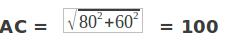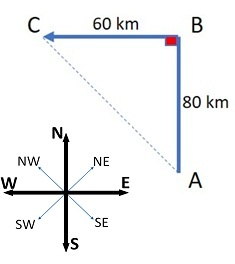• Some boys are sitting in three rows all facing North such that B is in the middle row. C is just to the right of B but in the same row.
• D is just behind of C while A is in the North of B.
In which direction of A is D?

a. South-East
b. South-West
c. North-West
d. North-East
 Answer  Explanation ANSWER: South-East Explanation: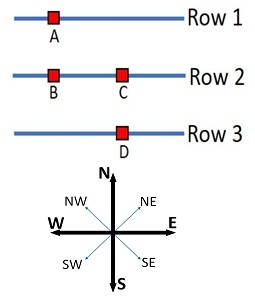1. P x Q means P is the father of Q
2. P - Q means P is the sister of Q
3. P + Q means P is the mother of Q
4. P ÷ Q means P is the brother of Q

In the expression, B + D x M ÷ N, how is M related to B?

a. Son
b. Grandson
c. Granddaughter
d. Daughter
 Answer  Explanation ANSWER: Grandson Explanation: B + D x M ÷ N means B is the mother of D who is the father of M, who, in turn, is the brother of N. Thus, M is the son of D, whose mother is B i.e. M is B’s grandson.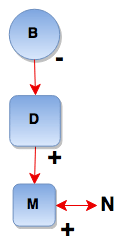1. P x Q means P is the father of Q
2. P - Q means P is the sister of Q
3. P + Q means P is the mother of Q
4. P ÷ Q means P is the brother of Q

Which of the following represents ‘J is the son of F’?

a. J ÷ R – T x F
b. J + R – T x F
c. J ÷ M – N x F
d. None of these
 Answer  Explanation ANSWER: None of these Explanation: J ÷ R – T x F means J is the brother of R who is the sister of T who is the father of F i.e. J is the uncle of F. J + R – T x F means J is the mother of R who is the sister of T who is the father of F i.e. J is the grandmother of FJ ÷ M – N x F means J is the brother of M who is the sister of N who is the father of F i.e. J is the uncle of FHere, none of the options follow.

18)   How much does a watch gain or lose per day, if its hands coincide every 64 minutes?

a. Gain 92 min
b. Lose 9 min
c. Lose 34 (5/11) min
d. Lose 32 (8/11) min
 Answer  Explanation ANSWER: Lose 32 (8/11) min Explanation: 55 min. spaces are covered in 60 min.60 min. spaces are covered in (60/55 × 60) min = 65 5/11 min Loss in 64 min = (65 5/11 - 64) = 16/11 minLoss in 24 hrs= (16/11 × 1/64 × 24 × 60) min = 32 (8/11) min.

19)   In the question given below consists of five or six statements followed by options consisting of three statements put together in a specific order. Choose the option which indicates a valid argument containing logically related statements that is, where the third statement is conclusion drawn from preceding two statements.

Statement - A. Cars are birds.
B. Some birds are ants.
C. Cars are ants.
D. Some Cars are ants.
E. Some Cars are not ants.
F. No car is ant.

a. ACE
c. BAC
d. BFD

20)   In the question given below consists of five or six statements followed by options consisting of three statements put together in a specific order. Choose the option which indicates a valid argument containing logically related statements that is, where the third statement is conclusion drawn from preceding two statements.

Statement - A. No apple is a door.
B. All poles are doors.
C. No pole is an apple.
D. Some poles are not apples.
E. Some apples are poles.
F. Some doors are not apples.

a. women
b. ACB
c. BDA
d. DFA
 Answer  Explanation ANSWER: women Explanation: No explanation is available for this question!

21)   The following question consists of some Problem Figures followed by other figures marked 1, 2, 3, 4 called the Answer Figures.

Find out the correct answer figure that should come next in the sequence of problem figures.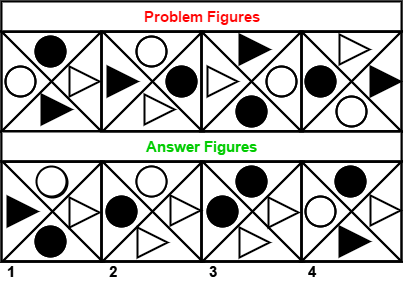a. 1
b. 2
c. 3
d. 4
 Answer  Explanation ANSWER: 4 Explanation: Elements are moved forward in each step in a set order, the preceding shallow element moves in place of filled element in clockwise order. The answer figure should be figure 4.

22)   The following question consists of some Problem Figures followed by other figures marked 1, 2, 3, 4 called the Answer Figures.

Find out the correct answer figure that should come next in the sequence of problem figures.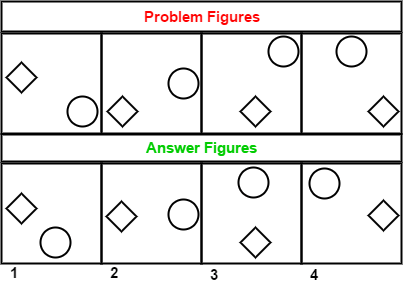a. 1
b. 2
c. 3
d. 4
 Answer  Explanation ANSWER: 4 Explanation: The circle and the diamond both move in anti-clockwise order along the sides of the square. The answer figure should be figure 4.